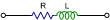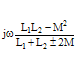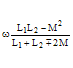# Impedance and Admittance Formulas for RLC Combinations

Here is an extensive table of impedance, admittance, magnitude, and phase angle equations (formulas) for fundamental series and parallel combinations of resistors, inductors, and capacitors. All schematics and equations assume ideal components, where resistors exhibit only resistance, capacitors exhibit only capacitance, and inductors exhibit only inductance.

For those unfamiliar with complex numbers, the "±j" operator signifies a phase of ±90°. Voltage across a capacitor lags the current through it by 90°, so -j is used along with its capacitive reactance (-j/ωC). Voltage across an inductor leads the current through it by 90°, so +j is used along with inductive reactance (jωL).

"M" is the mutual inductance between inductors.

"ω" is frequency in radians/second, and is equal to 2π times frequency in cycles/second.

This is probably one of the most comprehensive collections you will find on the Internet.

Z = R + jX           |Z| = (R2 + X2)½           ϕ = tan-1(X/R)           Y = 1/Z

 CircuitConfiguration ImpedanceZ = R + jX Magnitude{Z} = (R2 + X2)½ Phase Angleϕ = tan-1(X/R) AdmittanceY = 1/ZR R 0 1/RjωL ωL +π/2 -j/ωL-j/ωC 1/ωC -π/2 jωCjω(L1+L2±2M) ω(L1+L2±2M) +π/2 -j/[ω(L1+L2±2M)]-(j/ω)(1/C1+1/C2) (1/ω)(1/C1+1/C2) -π/2 jωC1C2/(C1+C2)R+jωL (R2+ω2L2)½ tan-1(ωL/R) (R-jωL)/(R2+ω2L2)R-j/ωC (1/ωC)(1+ω2C2R2)½ -tan-1(1/ωCR) (R+j/ωC)/(R2+1/ω2C2)j(ωL-1/ωC) (ωL-1/ωC) ±π/2 jωC/(1-ω2LC)R+j(ωL-1/ωC) [R2+(ωL-1/ωC)2]½ tan-1[(ωL-1/ωC)/R]R1R2/(R1+R2) R1R2/(R1+R2) 0 1/R1+1/R2+π/2-j/ω(C1+C2) 1/ω(C1+C2) -π/2 jω(C1+C2)ωLR/(R2+ω2L2)½ tan-1(R/ωL) 1/R-j/ωLR(1-jωCR)/(1+ω2C2R2) R/(1+ω2C2R2)½ -tan-1(ωCR) 1/R+jωCjωL/(1-ω2LC) ωL/(1-ω2LC) ±π/2 j(ωC-1/ωL)[(1/R)2+(ωC-1/ωL)2]-½ tan-1[R(1/ωL-ωC)] 1/R+j(ωC-1/ωL)Impedance ZMagnitude |Z|Phase Angle ϕAdmittanceImpedance ZMagnitude |Z|Phase Angle ϕAdmittanceImpedance ZMagnitude |Z|Phase Angle ϕAdmittanceImpedance ZMagnitude |Z|Phase Angle ϕAdmittanceImpedance ZMagnitude |Z|Phase Angle ϕ tan-1(X1/R1)+tan-1(X2/R2)-tan-1[(X1+X2)/(R1+R2)] Admittance 1/(R1+jX1)+1/(R2+jX2)

Note: Corrections made to RLC Magnitude and Admittance formulas, and to RL||R Admittance formula on 7/3/2014. Thanks to Bob N. for catching the errors.

(source: Reference Data for Engineers, 1993)About RF CafeCopyright: 1996 - 2024Webmaster:    Kirt Blattenberger,    BSEE - KB3UON RF Cafe began life in 1996 as "RF Tools" in an AOL screen name web space totaling 2 MB. Its primary purpose was to provide me with ready access to commonly needed formulas and reference material while performing my work as an RF system and circuit design engineer. The Internet was still largely an unknown entity at the time and not much was available in the form of WYSIWYG ... All trademarks, copyrights, patents, and other rights of ownership to images and text used on the RF Cafe website are hereby acknowledged. My Hobby Website:  AirplanesAndRockets.com